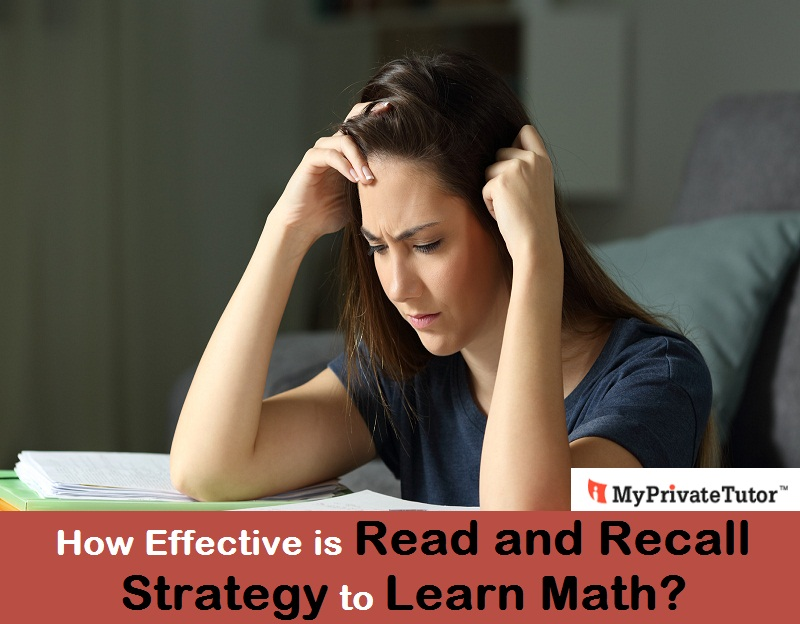# How Effective is Read and Recall Strategy to Learn Math?

Apr 07, 2021A number of students follow read and recall strategy for a better learning of any subject. Basically, read and recall is a systematic method to read a chapter and memorize what they have read. The learners here read a short paragraph and try to recall the same paragraph to ensure proper learning.

But the effectiveness of read and recall strategy to learn math is questionable because of few lessons. Also, there are debates among the educationists regarding the effectiveness of this learning method:

### Math Needs More Understanding

Read and recall technique is mainly for theory-based subjects. For Math, the way of exercising is a bit different because until the learners understand the mathematical concepts, recalling will be of no use. This is a reason why read and recall method seems to be the least effective strategy to learn math.

Now, students have the belief that the formulas of math and table are a few things that the students need to byheart. Here, read and recall strategy can be an effective one. But, it is believed that formulas can be recalled in easier ways if the students can understand the theory behind the formula.

(a + b)2 = a2 + 2ab +b2 is a very common formula in math. But until the students know that (a + b)2 = (a + b) * (a +b) = a2 + ab + ba + b2 = a2 + 2ab + b2

Just like this simple formula, all other mathematical formula have own explanation. Until that explanation is clear to the students, they will not be able to develop their math skills at all. Similarly, the application of the formula in complicated mathematical problems will be difficult to the learners. From this perspective, read and recall does not seem to be an effective strategy for learning math.

### Math - A Subject of Practice

Read and recall cannot assist in learning math because perfection can be achieved in this subject through practice. Regular practice of math will develop a habit in the students and they will be confident enough even during attempting difficult problems.

### Digging Deep = New Strategy

Math appears easy for those who never try to recall. Instead, they understand the method, dig deep into the problem and get smart strategies to solve these problems easily.
Students not necessarily need the tutorials to understand the smart tricks. Sometimes they need to practice a lot and dig deep into the problem. Also, they need to apply different methods to solve the same problem.

For example, students will learn some new formulas and methods in 7th standard, solving the problems of junior classes by applying these formulas can be a good exercise for the students. From this perspective too, read and recall don't seem to be much effective.

### Recalling the Tables

Now, tables are to be recalled for the benefit of the learners. To be more specific, recalling the tables will save much time for the learners during the exam. Larger calculations may include multiplication or division of large numbers. Here, recalling the tables is a really effective way. For this, they need to read the tables and recall those again and again.

### Read and Recall the Divisibility Rules

Application of divisibility rules often makes mathematical problems easier. So, in case the students read and recall the divisibility rules, it will be their benefit only. Until and unless the students read and recall the divisibility rules, it will not be possible for them to figure out the equations shortly. It will take much time to use the descriptive method and find out the answer. Especially during the exams, read and recall technique is really good for the students to finish the paper on time. Recalling these will be a great help to slow learners

### Recalling the Theorems

Theorems are backed by valid reason and explanation. Even after understanding the description, reading and recalling the theorems is a good idea to perform well in the exam. Exams have a specific time and there are several questions to attempt. Now, recalling the theorems will nonetheless be an advantage to them.

### Conclusion

It cannot be specifically said that read and recall techniques are good or bad to learn math. For a few parts or chapters, it is a good idea to use read and recall, but for most of the chapters, a thorough understanding of concepts and theories is mandatory. Math Tutors may help here. So, the students can definitely use this learning method to speed up during the exam. But proper understanding and practice will help the students to recall the lessons as well.

### Summary

To learn math faster, many studnets use the read and recall strategy. Check whether it helps in math learning or not.

Article Posted in: Academics Maths Student Tips### Rachita

With a passion for writing, Rachita is in the field of content writing for more than 5 years. Here, she writes the blogs for MyPrivateTutor Singapore. Being a literature student, she has a strong addiction to reading as well. On a lazy day, she just needs a cozy setting and her favorite book or web series.